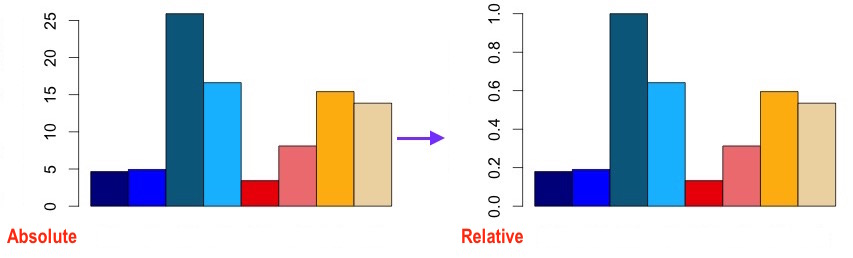## R: row values to relative and batch plotting

Actually there is a very simple solution without need to search for specific functions. But it did not come to my mind in the past several days, when I tried to use `apply` and its variants and to set up `function` or a loop inside the rows and columns. The idea is to set the maxmium value in each row to 1 and the rest values scaled.

Now comes the easiest way.

#### 1. To make a single plot

Assume that you have a matrix called ‘mx’ and you want to make a barplot for a specific row “gene_id_1”

 ``````1 2 3 `````` ``````idx<-"gene_id_1" barplot(mx[idx, ]/max(mx[idx,]), col = colo3, space = 0, main = idx, ylab="Relative expression") ``````So the keypoint is `mx[idx, ]/max(mx[idx,]`, which divides each row element by the largest one in this row.

#### 2. To plot all rows and make each row a single barplot

A `for` loop just did the right thing.

 ``````1 2 3 4 5 6 `````` ``````i<-1; for (i in 1:length(mx)) { pdf(paste(i,".pdf", sep="")); barplot(mx[i, ]/max(mx[i, ]), col = colo3, space = 0, ylab="Relative expression"); dev.off() } ``````

#### 3. To define rows from a file (or input) and make batch plotting

 ``````1 2 `````` ``````idx<-readLines(file("stdin"),1) GI<-scan(file=idx, what=character()); ``````
And use `mx[GI[i],]/max(mx[GI[i],])`.